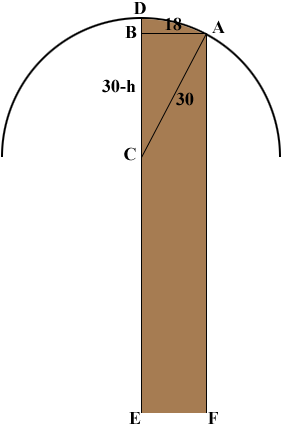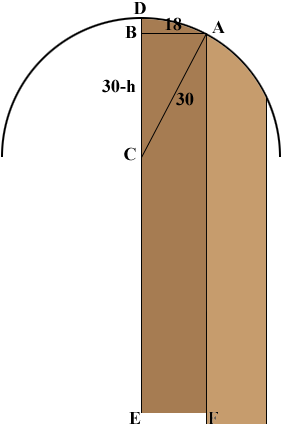SEARCH HOMEMath Central Quandaries & QueriesI work for a shutter company and am in need of a formula to figure out what the low leg height would be if given the width of shutter, the high point of arch top and the radius. example would be a 18" wide shutter with a 80" high leg on the right side and a 30" radius. I would need a way to figure what the low leg height (left side of shutter) would be. Or if given width, low leg height and radius what the high side would be? If any of this can be given in laymen's terms it would be much appreciated. Thanks, BrianBrian,

I'll try this but I'm not sure I understand the situation. My interpretation is that you have an 18 inch wide vertical shutter with the top cut on a circular arc with the long side of the shutter 80 inches long and you want to know the length of the short side.

I drew a diagram (not to scale) with the long side of the shutter at the peak of the arch.If the distance from D to B is h inches then Pythagoras theorem applied to triangle ABC yields

(30 - h)2 + 182 = 302.

Expanding this equation and solving for h gives h = 6 inches. Hence the short side of the shutter is 6 inches shorter than the long side so it is 74 inches long.

The complication here is that if the shutter is at a different position along the arc, for example as in the diagram below with the long side meeting the arc at A, then the decrease in the length of the short side is much more than 6 inches as is evident in the diagram.Let me know if I have misinterpreted your question,
HarleyMath Central is supported by the University of Regina and The Pacific Institute for the Mathematical Sciences.